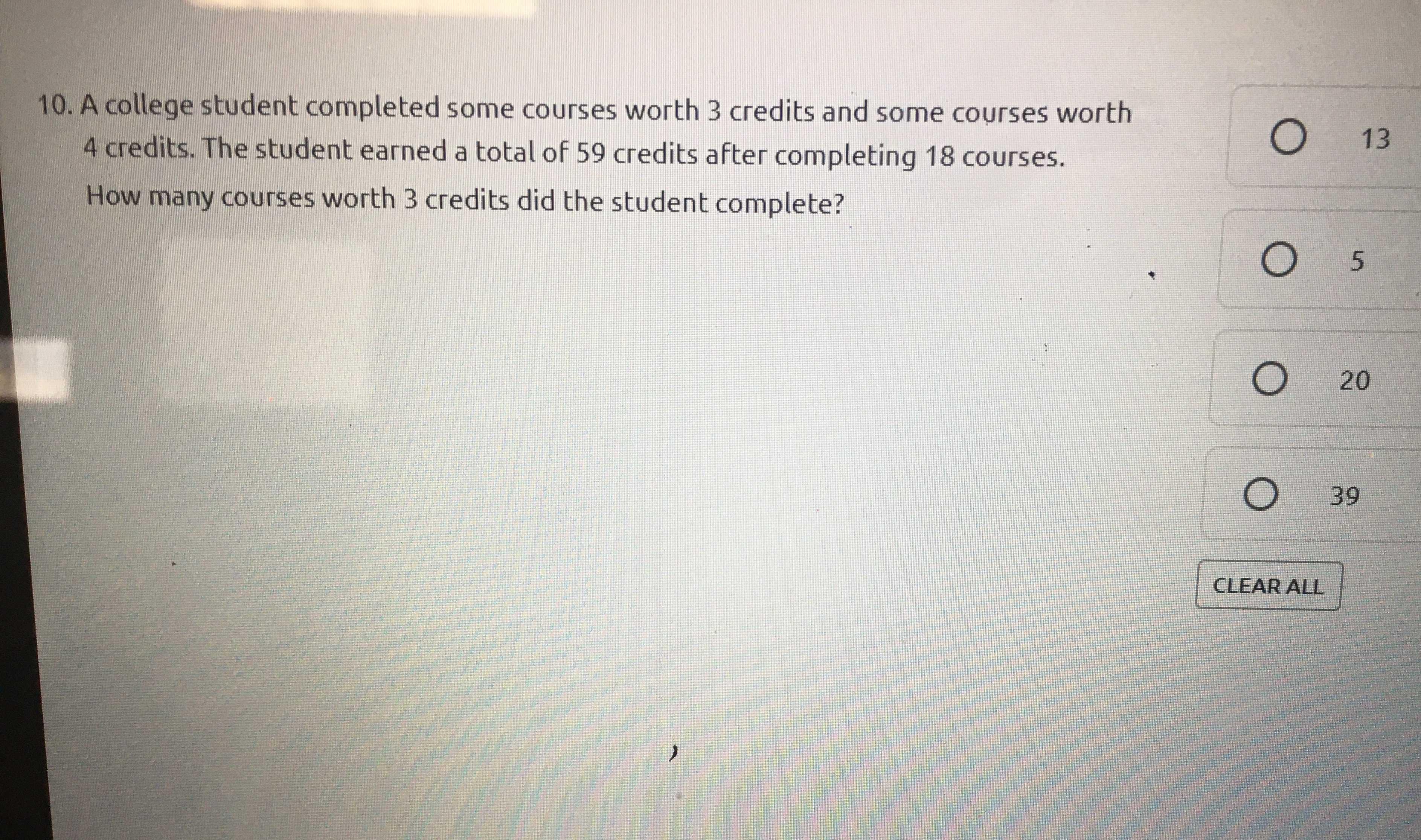### Still have math questions?

Arithmetic
Question10. A college student completed some courses worth $$3$$ credits and some courses worth

$$4$$ credits. The student earned a total of $$59$$ credits after completing $$18$$ courses. How many courses worth $$3$$ credits did the student complete?